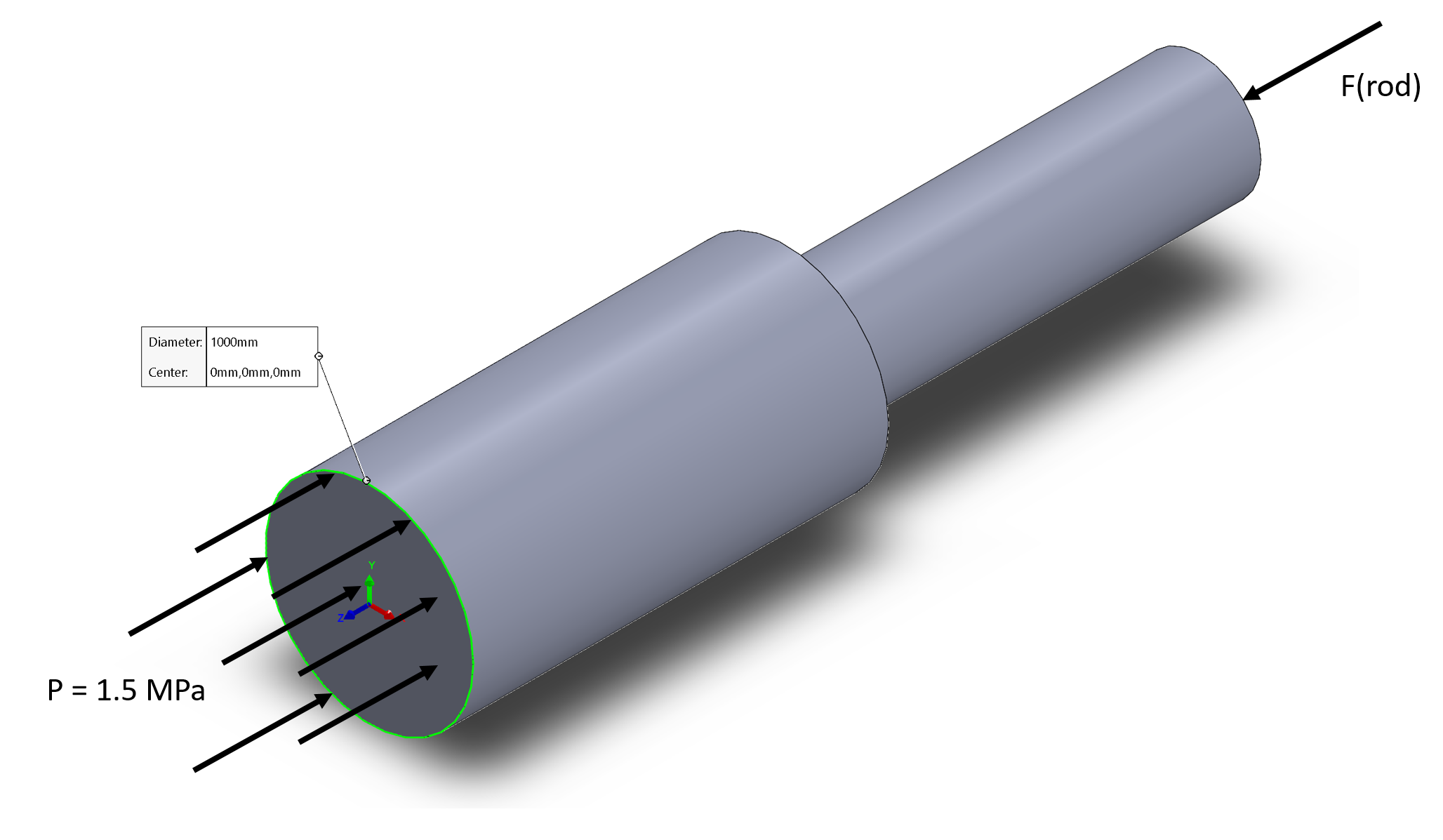## A Piston's Cross Section

A steam engine's piston has a 1,000 mm diameter, and is subject to a max 1.5 MPa pressure force (P). What is the piston's cross sectional area if the rod portion has a 70 MPa design stress?Hint
$$\sum F=Pressure\cdot Area=F_{rod}$$$$$Area_{rod}=\frac{F_{rod}}{\sigma }$$$
Given how pistons operate, the force from the smaller rod must equal to the pressure force on the opposite end. Therefore:
$$\sum F=Pressure\cdot Area=F_{rod}$$$$$F_{rod}=(1.5\times 10^{6}Pa)\left ( \frac{\pi (1m)^{2}}{4} \right )=1,177.5 kN$$$
Now that we solved for the rod's force and the design stress was given in the problem statement, we can work backwards to find the associated area.
$$Area_{rod}=\frac{F_{rod}}{\sigma }=\frac{1,177,500 N}{70\cdot 10^{6}Pa}$$$$$Area_{rod}=0.017 \:m^2$$$
$$Area_{rod}=0.017 \:m^2$$\$